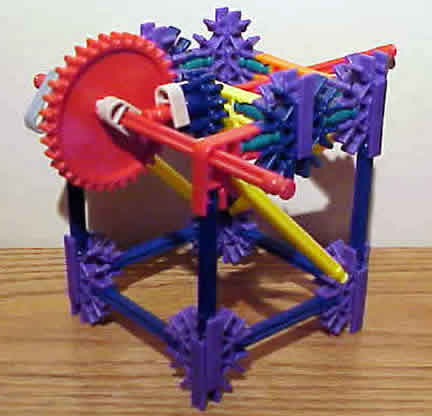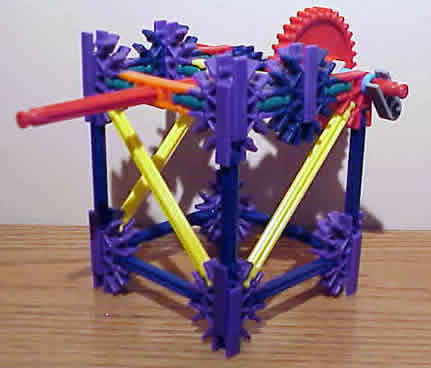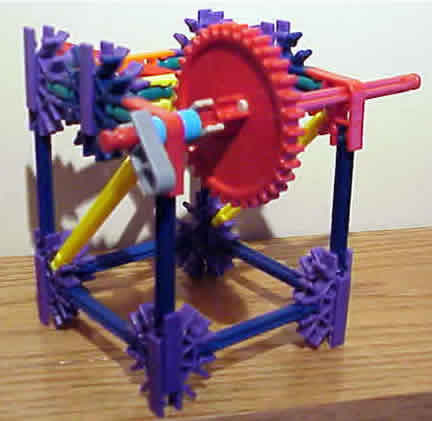# Gears

## Exploration

### Focus question

• What is the relationship between the number of gears and how many times two gears turn?
• How does the force change with the radius of a gear?
• What other relationship can you determine about gears?

### Procedure for gears of same size.

Focus Question

How does a system with two gears of the same size operate?

Suggestions

• Look at the pictures below to make a two gear system.
• May want to look at pictures of the mass hangers to see how they can be attached to the axles. (weight/mass hanger)
• Questions In what direction does each gear turn? CW (clockwise) or CCW (counter clockwise)?
• If one gear rotates once, how many times does the other gear rotate?
• How many teeth are on each gear?
• How does that relate to the rotation of the gears?
• How does the size of the gear change the rate that the axles and gears turn?
• If weights (washers) are connected to the axles (weight/mass hanger) how many are needed to lift 1 washer on the other axle? 5, 10, 15 washers?
• Is there a difference between lifting with the larger or smaller?
• Why is there a difference or not?
• If weights (washers) are connected to one axle and a spring scale to measure force to the other, how much force is needed to lift 1, 5, 10, 15, 20 washers?
• What does the relationship of the above data look like on a graph?

### Procedure for gears of same size

Focus Question

How does a system with two different sized gears operate?

Suggestions

• Look at the pictures below to make a two gear system.
• May want to look at pictures of the mass hangers to see how they can be attached to the axles. (weight/mass hanger)
• Questions In what direction does each gear turn? CW (clockwise) or CCW (counter clockwise)?
• If one gear rotates once, how many times does the other gear rotate?
• How many teeth are on each gear?
• How does that relate to the rotation of the gears?
• How does the size of the gear change the rate that the axles and gears turn?
• If weights (washers) are connected to the axles (weight/mass hanger) how many are needed to lift 1 washer on the other axle? 5, 10, 15 washers?
• Is there a difference between lifting with the larger or smaller?
• Why is there a difference or not?
• If weights (washers) are connected to one axle and a spring scale to measure force to the other, how much force is needed to lift 1, 5, 10, 15, 20 washers?
• What does the relationship of the above data look like on a graph?

Three groups of photos: gear box with gears the same size, gear box with large and small gear, & gears with smaller different sizes.

## Gear photo album

#### Gears same size and weight hangers - eight views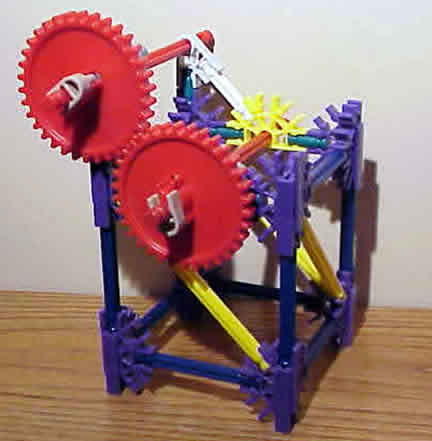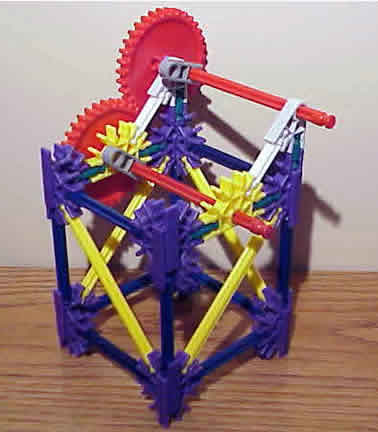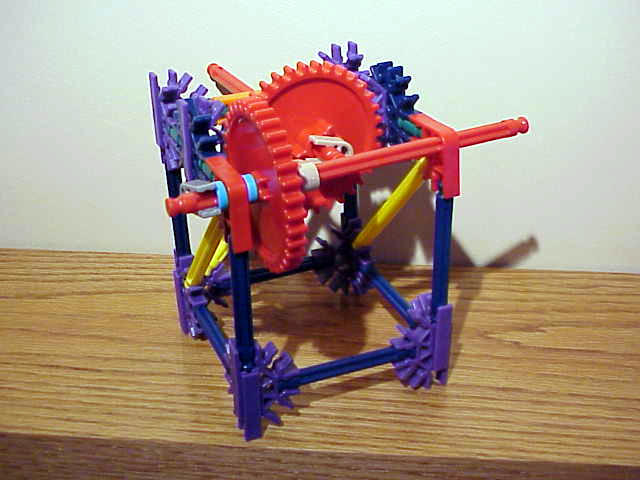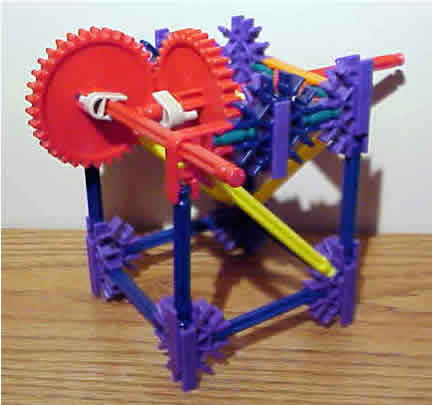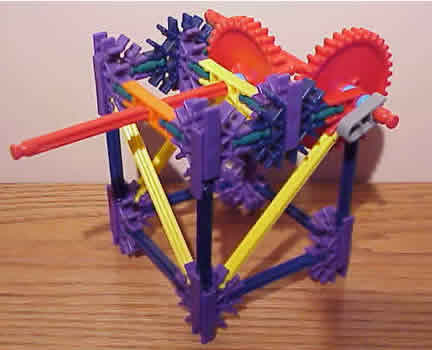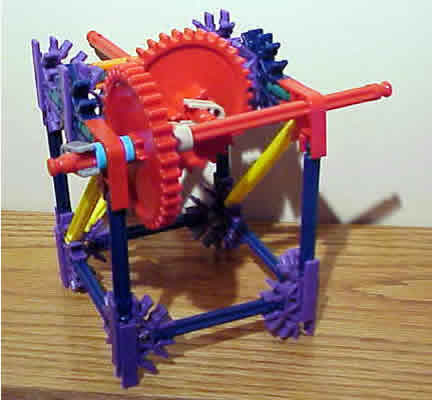#### Large gear small gear - 5 views of same system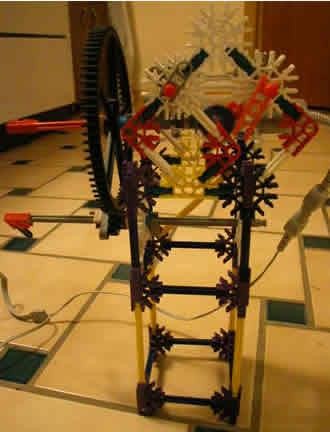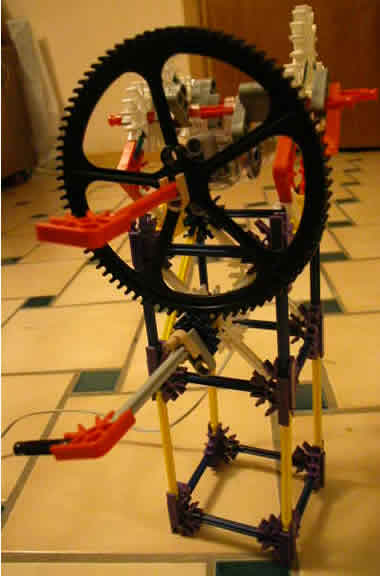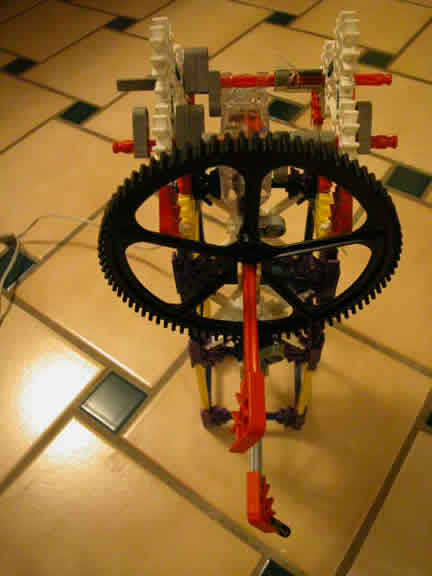#### Smaller gears two different sizes (red larger blue smaller)